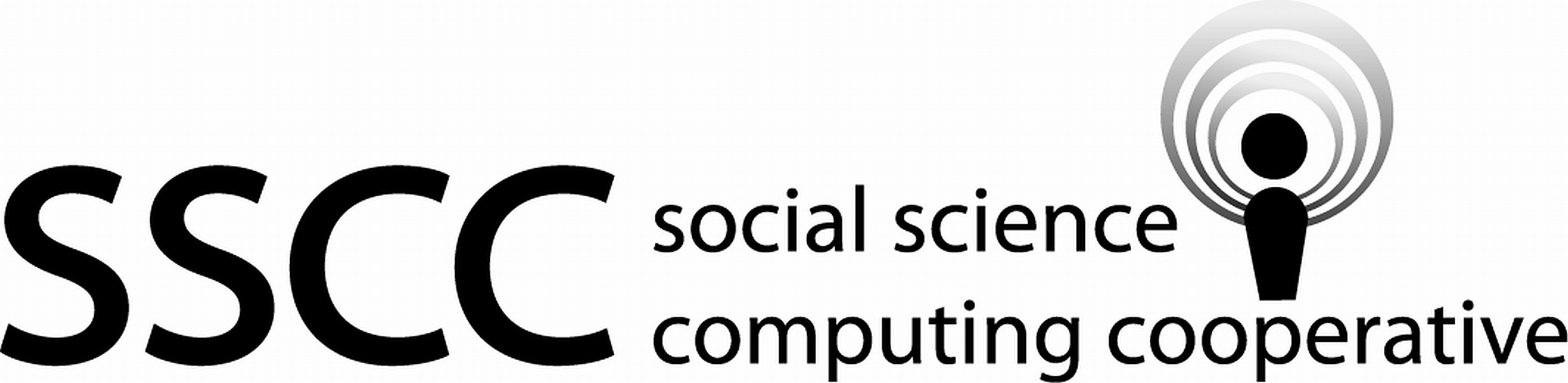Supporting Statistical Analysis for Research

## 2.3 The pipe operator

The pipe operator, %>%, passes an object to a function as the first parameter. The function call,

    function_name(data_object, other_parameters)

becomes,

    data_object %>% function_name(other_parameters)

With the pipe operator.

The pipe operator reduces the coding load of saving intermediate results that will only be referencing in next line of code. This reduction in managing intermediate results can make your code easier to read.

### 2.3.1 Examples

1. Base R

The following code creates a vector of 15 numeric values. This vector is then rounded to two significant digits, sorted in descending order, and then head() displays a few of the largest values.

set.seed(749875)
number_data <- runif(n = 15, min = 0, max = 1000)

head(sort(round(number_data, digit = 2), decreasing = TRUE))
 997.62 813.26 797.96 733.98 732.67 675.45

To read the above base R code, one reads from the inner most parenthises to the outer most. This nesting of functions can make reading base R code challenging.

Another base R approach that avoids deeply nesting functions is to save the intermediate results. The intermediate results are then used in the next function as a separate command.

number_round <- round(number_data, digit = 2)
number_sort <- sort(number_round, decreasing = TRUE)
head(number_sort)
 997.62 813.26 797.96 733.98 732.67 675.45

This is also a more natural order of the functions. It does require the intermediate results to be saved. These intermediate results are only used by the function on the next line.

2. Using the pipe operator

The pipe operator allow the order of the data and functions to more closely match the order they are evaluated, without needing to save the intermediate results.

number_data %>%
round(digits = 2) %>%
sort(decreasing = TRUE) %>%
head()
 997.62 813.26 797.96 733.98 732.67 675.45

This coding style places the most important information about what is being operated on and the operations that are being done on the left side of the page. The details of what is being done are found further to the right on the page. This is considered easier to read code.